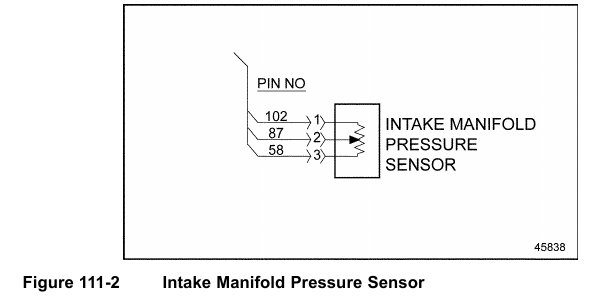# Series 60 – SPN 3563/FMI 4 – INTAKE MANIFOLD PRESSURE OUTSIDE NORMAL RANGE

The typical diagnosis for this fault is an Intake Manifold Pressure Sensor open circuit.

111.4.1 Open Circuit Check
Check as follows:
1. Disconnect the Intake Manifold Pressure Sensor (IMP Sensor).
2. Measure the resistance between pin 1 and 3 of the IMP Sensor.
[a] If resistance is greater than 130 kΩ, replace the IMP Sensor. Refer to section
111.4.1.1.
[b] If resistance is less than 130 kΩ, go to step 3.
3. Measure the resistance between pins 1 and 2 of the sensor. See Figure 111-2.[a] If resistance is greater than 130 kΩ, replace the IMP Sensor. Refer to section
111.4.1.1.
[b] If resistance is less than 130 kΩ, go to step 4.
4. Turn the ignition switch to the ON position.
5. Measure the voltage between pins 1 and 3 of the IMP Sensor harness connector.
[a] If the voltage is between 4.5 and 5.5 volts, go to step 7.
[b] If the voltage is less than 4.5 volts, go to step 6.
6. Measure the voltage between pin 3 of the IMP Sensor harness connector and ground.
[a] If the voltage is between 4.5 and 5.5 volts, repair the open circuit between pin 1 of
the IMP Sensor harness connector and pin 105 of the 120–pin MCM connector.
Refer to section 111.4.1.1.
[b] If the voltage is less than 4.5 volts, repair the open circuit between pin 3 of
the IMP Sensor harness connector and pin 82 of the 120–pin MCM connector.
Refer to section 111.4.1.1.
7. Turn the ignition OFF.
8. Disconnect the 120–pin MCM connector.
9. Measure the resistance between pins 1 and 3 of the IMP Sensor harness connector.
[a] If the resistance is greater than 5 Ω, go to step 10.
[b] If the resistance is less than 5 Ω, repair the short between pins 105 and 82 of the
120–pin MCM connector. Refer to section 111.4.1.1.
10. Measure the resistance between pins 1 and 2 of the IMP Sensor harness connector.
[a] If the resistance is greater than 5 Ω, go to step 11.
[b] If the resistance is less than 5 Ω, repair the short between pins 105 and 54 of the
120–pin MCM connector. Refer to section 111.4.1.1.
11. Measure the resistance between pin 3 of the IMP Sensor harness connector and ground.
[a] If the resistance is greater than 5 Ω, go to step 12.
[b] If the resistance is less than 5 Ω, repair the short between pin 82 of the 120–pin
MCM connector and ground. Refer to section 111.4.1.1.
12. Measure the resistance between pin 2 of the IMP Sensor harness connector and ground.
[a] If the resistance is greater than 5 Ω, repair the open circuit between pin 2 of
the IMP Sensor harness connector and pin 54 of the 120–pin MCM connector.
Refer to section 111.4.1.1.
[b] If the resistance is less than 5 Ω, repair the short between pin 54 of the 120–pin MCM
connector. Refer to section 111.4.1.1.

111.4.1.1 Verify Repairs
Verify repairs as follows:
1. Turn ignition OFF.
2. Reconnect any electrical connections that were disconnected to perform the diagnosis.